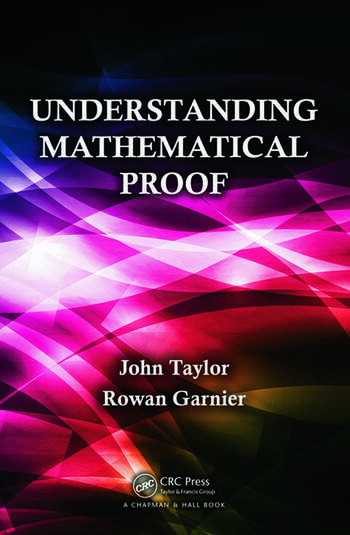Understanding Mathematical Proof

1st Edition

John Taylor, Rowan Garnier

Chapman and Hall/CRC
Published March 21, 2014
Textbook - 414 Pages - 62 B/W Illustrations
ISBN 9781466514904 - CAT# K15018

For Instructors Request Inspection Copy

was \$70.95

USD\$56.76

SAVE ~\$14.19

FREE Standard Shipping!

Preview

Summary

The notion of proof is central to mathematics yet it is one of the most difficult aspects of the subject to teach and master. In particular, undergraduate mathematics students often experience difficulties in understanding and constructing proofs.

Understanding Mathematical Proof describes the nature of mathematical proof, explores the various techniques that mathematicians adopt to prove their results, and offers advice and strategies for constructing proofs. It will improve students’ ability to understand proofs and construct correct proofs of their own.

The first chapter of the text introduces the kind of reasoning that mathematicians use when writing their proofs and gives some example proofs to set the scene. The book then describes basic logic to enable an understanding of the structure of both individual mathematical statements and whole mathematical proofs. It also explains the notions of sets and functions and dissects several proofs with a view to exposing some of the underlying features common to most mathematical proofs. The remainder of the book delves further into different types of proof, including direct proof, proof using contrapositive, proof by contradiction, and mathematical induction. The authors also discuss existence and uniqueness proofs and the role of counter examples.

Instructors

We provide complimentary e-inspection copies of primary textbooks to instructors considering our books for course adoption.